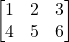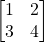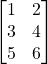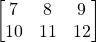# Chapter 4

## Chapter 4 Code Examples

### MATLAB Matrix Examples

Matrices from Equations 4.1 and 4.2

A = [1,2,3;4,5,6]
B = [10,11,12;13,14,15]

Matrices from Equations 4.7 and 4.8

A = [1,1,3;2,1,0]
B = [1,2;0,2;2,1]

### Python Matrix Examples

Matrices from Equations 4.1 and 4.2

A = np.array([[1,2,3],[4,5,6]])
B = n

Matrices from Equations 4.7 and 4.8

A = np.array([[1,1,3],[2,1,0]])
B = np.array([[1,2],[0,2],[2,1]])

## Exercises

Given matrices:
A=B=C=D=Calculate the following:

1. A – C’
2. C’ + 3D
3. BA
4. CB
5.6. AA’
7. D’D